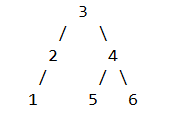# Josh Technology Interview Experience for SDE (Off-Campus)

• Difficulty Level : Medium
• Last Updated : 12 Jan, 2021

Online Selection Test: The comprised of three phases and all of them were on the same day.

• Phase 1(MCQ ROUND): This round consists of 50 MCQs on C programming language, we were given 50 minutes to solve 50 questions, this phase also had some output based ques where we were given a code snippet, and we were supposed to type the output of that program. Output-based questions didn’t have negative marking where the MCQ questions had negative marking, if you attempt around 30 questions you will be shortlisted for the next round.
• Phase 2(Subjective Round): This round had 3 coding questions and one output based question, they mainly focus on trees so if you want to crack Josh do practice a lot of questions based on tree
Questions:
1. Given a number represented as the linked list, we were supposed to multiply this number by 4.
2. Find the length of the longest alternating even-odd subarray
3. Given a tree, we need to return a list representing the minimum number that should be added at each level such that the sum of the nodes at each level form an AP
4. It was a C output based ques based on  trees
• Phase 3(Coding Round): This round had three coding questions, and they were quite easy
1. Buy-Sell Stocks to maximize the profit It is a standard DP problem
2. Given a linked list were supposed to update the value of each node by the sum of the node itself and the sum of (n-k)th node
`e.g. 31->2->3->4->5->11->50  Output :  42->13->8->4->8->13->42`
3. Given a tree we need to check if the tree was balanced or not ie |height of left subtree – the height of right subtree| <= 1

Technical Rounds: I directly had my second interview after the online test while many people had their first technical interview as well, all the rounds were completely focused on trees

Interview Round 2: In this round, three questions were asked and all of them were based on trees

• Given a tree were each leaf to root path represents a number, and we were supposed to return the sum of all the numbers that were formed int the treein its case number formed are 123,543 and 643 and the final output of the code should be 123+543+643=1309  in O(N) time and O(1) space

Interview Round 3:

• Given a BST and a target value we need to split the BST into two BST such that all the nodes smaller than or equal to the target value lie in the first BST while others lie in the second BST in O(logn) time and O(1) space
• Given an anti-BST and a target value we were expected to return the sum of all the nodes that are at K distance away from the target value in O(1) space
• A recent project that I have worked upon

HR Round:

1. How was the experience so far
2. What was the level of difficulty
3. How did you prepare for the rounds
4. Who you are as a person